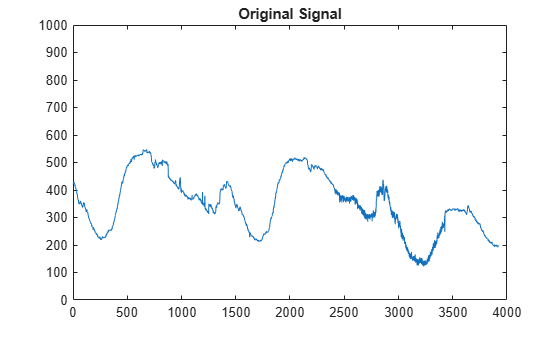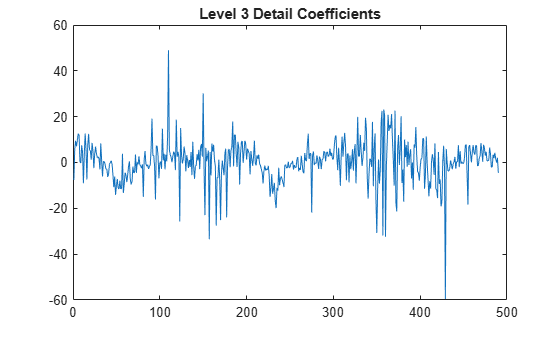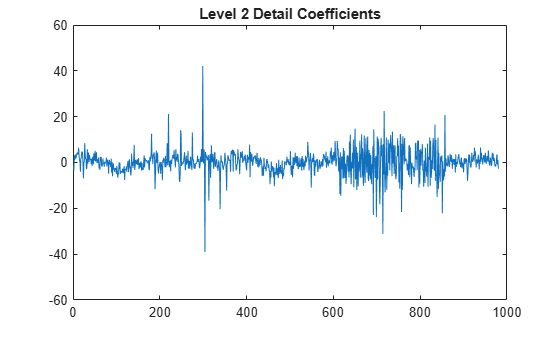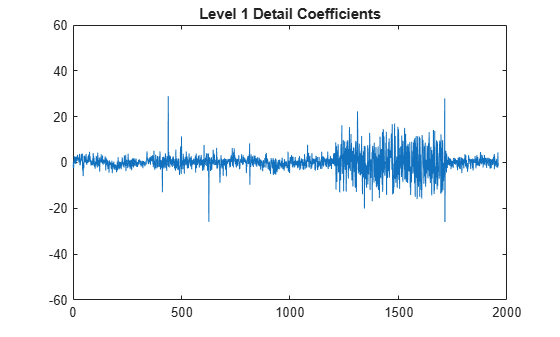# detcoef

1-D detail coefficients

## Syntax

``D = detcoef(C,L)``
``D = detcoef(C,L,N)``
``D = detcoef(C,L,N,'cells')``
``[D1,…,Dp] = detcoef(C,L,N)``

## Description

````D = detcoef(C,L)` extracts the detail coefficients at the coarsest scale from the wavelet decomposition structure `[C, L]`. See `wavedec` for more information on `C` and `L`.```
````D = detcoef(C,L,N)` extracts the detail coefficients at the level or levels specified by `N`.```
````D = detcoef(C,L,N,'cells')` returns a cell array containing the detail coefficients. A minimum of two levels must be specified. The ith element of `D` contains the detail coefficients at the ith specified level. If `length(N)>1`, the ```D = detcoef(C,L,N)``` is equivalent to ```D = detcoef(C,L,N,'cells')```.`D = detcoef(C,L,'cells')` is equivalent to `D = detcoef(C,L,[1:NMAX])`, where `NMAX = length(L)-2`. ```

example

````[D1,…,Dp] = detcoef(C,L,N)` extracts the detail coefficients at the levels specified by `N`. The length of `N` must equal the number of output arguments.```

## Examples

collapse all

This example shows how to obtain and plot the detail coefficients for an electrical current signal. This example uses zero-padding (see `dwtmode`).

Load the signal and select the first 3920 samples.

```origmode = dwtmode('status','nodisplay'); dwtmode('zpd','nodisplay') load leleccum; s = leleccum(1:3920);```

Perform the decomposition at level 3 using `db1`. Extract the detail coefficients at levels 1, 2, and 3 from the decomposition structure.

```[c,l] = wavedec(s,3,'db1'); [cd1,cd2,cd3] = detcoef(c,l,[1 2 3]);```

Plot the original signal.

```plot(s) title('Original signal') ylim([0 1000])```Plot the level 3 detail coefficients.

```plot(cd3) title('Level 3 detail coefficients (cd3)') ylim([-60 60])```Plot the level 2 detail coefficients.

```plot (cd2) title('Level 2 detail coefficients (cd2)') ylim([-60 60])```Plot the level 1 detail coefficients.

```plot (cd1) title('Level 1 detail coefficients (cd1)') ylim([-60 60])```Restore the original extension mode.

`dwtmode(origmode,'nodisplay')`

## Input Arguments

collapse all

Wavelet decomposition vector, specified as a real-valued vector. The vector `C` is the output of `wavedec`.

Data Types: `single` | `double`
Complex Number Support: Yes

Bookkeeping vector, specified as a vector of positive integers. The bookkeeping vector `L` contains the number of coefficients by level. The bookkeeping vector is used to parse the coefficients in the wavelet decomposition vector `C`. The vectors `C` and `L` are the outputs of `wavedec`.

Data Types: `single` | `double`

Detail level to extract from the wavelet decomposition, specified as a positive integer or a vector of positive integers.

• If `N` is an integer, then `N` must be an integer such that `1 ≤ N ≤ NMAX`, where ```NMAX = length(L)-2```.

• If `N` is a vector of integers, then `N(j)` must be an integer such that `1 ≤ N(j) ≤ NMAX`, where ```j = 1,…,length(N)```.

## Output Arguments

collapse all

Detail coefficients, returned as a real-valued vector or a cell array. If `D` is a cell array, the ith element of `D` are the detail coefficients at the level specified by the ith element of `N`.

Detail coefficients, returned as set of real-valued vectors. The ith output argument are the detail coefficients at the level specified by the corresponding element of `N`.

## Extended Capabilities

### C/C++ Code GenerationGenerate C and C++ code using MATLAB® Coder™.